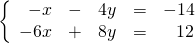Chapter 5: Systems of Equations

# 5.2 Substitution Solutions

While solving a system by graphing has advantages, it also has several limitations. First, it requires the graph to be perfectly drawn: if the lines are not straight, it may result in the wrong answer. Second, graphing is challenging if the values are really large—over 100, for example—or if the answer is a decimal that the graph will not be able to depict accurately, like 3.2134. For these reasons, graphing is rarely used to solve systems of equations. Commonly, algebraic approaches such as substitution are used instead.

Example 5.2.1

Find the intersection of the equations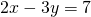and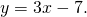Since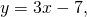substitute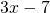for the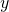in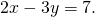The result of this looks like: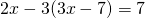Now solve for the variable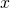: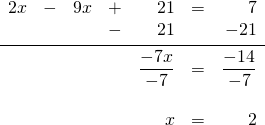Once the-coordinate is known, the-coordinate is easily found.

To find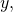use the equations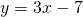and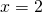: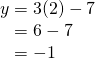These lines intersect atand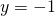, or at the coordinate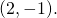This means the intersection is both consistent and independent.

Example 5.2.2

Find the intersection of the equations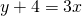and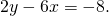To solve this using substitution,ormust be isolated. The first equation is the easiest in which to isolate a variable: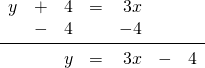Substituting this value forinto the second equation yields: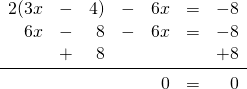The equations are identical, and when they are combined, they completely cancel out. This is an example of a consistent and dependent set of equations that has many solutions.

Example 5.2.3

Find the intersection of the equations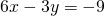and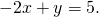The second equation looks to be the easiest in which to isolate a variable, so: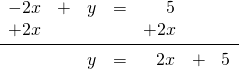Substituting this into the first equation yields: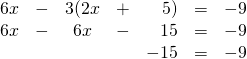The variables cancel out, resulting in an untrue statement. These are parallel lines that have identical variables but different intercepts. There is no solution, and these are inconsistent equations.

# Questions

For questions 1 to 20, solve each system of equations by substitution.

1.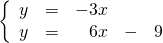2.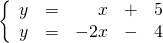3.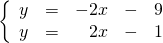4.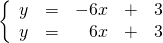5.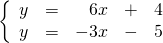6.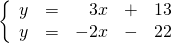7.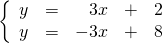8.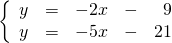9.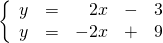10.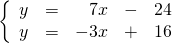11.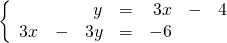12.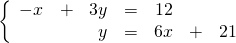13.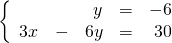14.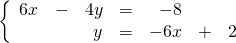15.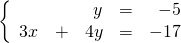16.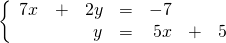17.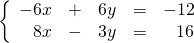18.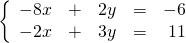19.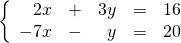20.